# Lookup The Second, Third, Or Nth Value in Excel

• Last Updated : 20 May, 2021

Excel is all about data management, data utilization. However, many times in Excel datasets, you must have noticed, that an argument is having 2, or more than 2 values. So, to look upon(or to use only the first assigned value), we use functions like VLOOKUP, INDEX.

However, in real-life situations, you may want to peek(use)  2nd,3rd, or even generalizing it to Nth value. So, to do this, we have two methods to do peeking on more than 1st order values.

These methods are:

1. Helper Formula
2. Array Formula

Let’s have a look at 1st method.

### HELPER METHOD: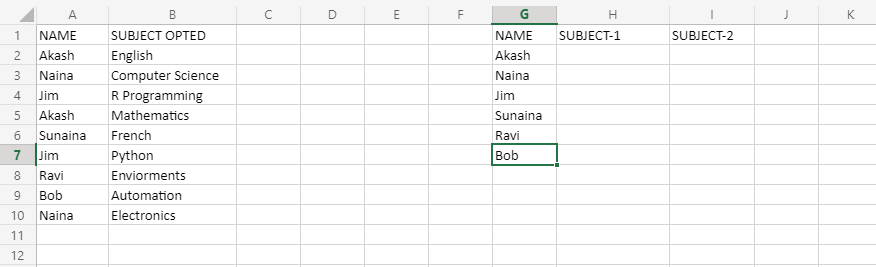For the purpose of understanding, we will be seeing the above dataset. In columns A and B, the enrollment subjects are given, but if you look closely, Akash and Naina, and Jim are enrolled in more than 1 subject. In columns G, H, I: the entries are done, but this time we have to fill data(ie subjects opted by students)in a horizontal manner. So, if we use methods like VLOOKUP and INDEX, the output will be different than expected, ie they will pick up the only first value of repeating arguments, For example, if we apply VLOOKUP OR INDEX function, it will only fill English as his subject, which is wrong. So, we need to upgrade this to 2nd,3rd, or even to Nth value peeking.

So, to do it, insert a blank column, just before that column, that contains repeated values of arguments.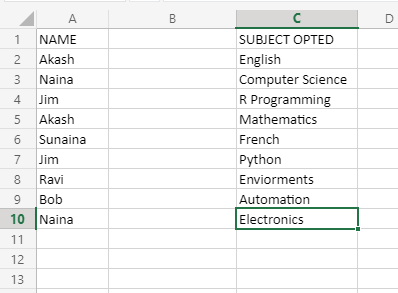In cell B2,write the following formula-

`=A2&COUNTIF(\$A\$2:\$A2,A2)`

The ‘=’ gives an indication to excel that everything ahead is a formula, and needs to be evaluated. Now, what this function will do? It will basically term each argument(which here is column NAME), will give unique and increasing numbers to each argument, so it will look like: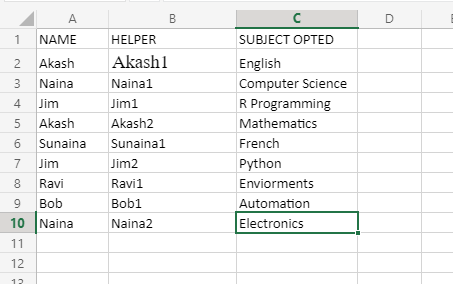Here, we gave every argument for each unique increase using COUNTIF in the above formula, and embedded it into Cell B, using the above formula.

Now, in cell H2,embed the following formula-

`=IFNA(VLOOKUP(\$G2&COLUMNS(\$H\$1:H1),\$B\$2:\$C\$10,2,0),"")`

The output will be like this: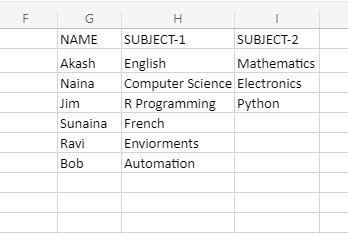Here \$G2&COLUMNS(\$H\$1:H1) is lookup or peek value finder for multiple values.

### ARRAY METHOD:

Now, this method helps you very much, when your requirement is to not change or alter the original dataset, such as by adding some additional(helper)columns.

Reminder-To execute array contained formulas, you need to press Ctrl+Shift+Enter keys, rather than pressing only Enter key.

We will be considering the same dataset, as used in the helper column example.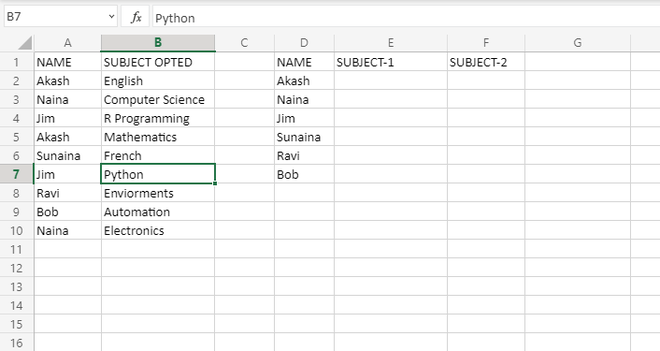Now, we will consider the formula, and understand it part by part, that how the formula works–

`=IFERROR(INDEX(\$B\$2:\$B\$10,SMALL(IF(\$A\$2:\$A\$10=\$D2,ROW(\$A\$2:\$A\$10)-1,""),COLUMNS(\$E\$1:E1))),"")`

This formula will get pasted in the E2 column.

Now, breaking the formula into parts, we will understand \$A\$2:\$A\$10=\$D2.

This formula is comparing in a range, which we provided as A2 to A10, with comparing it with D2 onwards.

The array returned by this will contain TRUE or FALSE values. The array returned, when this formula is applied on cell E2, will be:

`{TRUE;FALSE;FALSE;TRUE;FALSE;FALSE;FALSE;FALSE;FALSE}`

Here. counting of argument “Akash” is done. Look closely upon the returned array, wherever the name Akash is there the output is TRUE. For example, we put a range of comparing as from A2 to A10, so in this column range,1st,4th places are filled by argument Akash.

Now the second important part of this formula is:

`IF(\$A\$2:\$A\$10=\$D2, ROW(\$A\$2:\$A\$10)-1,""`

What this part is doing, is basically a decoration of the above formula, this formula will also print an array, but this time, whenever the required argument is matched, the TRUE value will not be printed, rather than the position of occurrence of that argument will be printed, and for FALSE values, we are printing just a “”, so for above example, where we were searching for argument Akash, the array in this format will be–

`{1;"";"";"";4;"";"";"";""}`

Now,the third important part of this formula is:

`SMALL(IF(\$A\$2:\$A\$10=\$D2,ROW(\$A\$2:\$A\$10)-1,""),COLUMNS(\$E\$1:E1)))`

Now, this function will pick up the first smallest, the second smallest, and so on, if you have more than 2 occurrences, and it will be placing them in the correct column, so for example, in our right table of the dataset, in cell E2, the value 1 will be returned by both COLUMN FUNCTION and SMALL FUNCTION. But, in column F2, the COLUMN FORMULA will return value 2, but the SMALL function will return value as 4 because it is the 2nd occurrence value for Akash.

Now, the third important part of this formula is:

`(INDEX(\$B\$2:\$B\$10,SMALL(IF(\$A\$2:\$A\$10=\$D2,ROW(\$A\$2:\$A\$10)-1,""),COLUMNS(\$E\$1:E1)))`

Now, the INDEX value is again a value returning function like COLUMN AND SMALL. But the different thing about INDEX value is that it will return the corresponding value of that argument, filled in column B2, So, for example, in column E2 it will fill value English and in cell F2, it will value Mathematics.

The IFERROR function will print a blank space, in case the INDEX function is unable to print a value.

The output of the array method will remain the same, just that you won’t need to add any extra columns and apply the formulas accordingly.

My Personal Notes arrow_drop_up